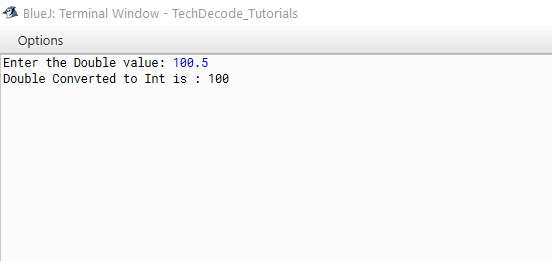# How to convert Double to Int in Java

The primitive data type in Java is represented by the int keyword. It’s where variables are declared. It can also be used with methods that return values of the integer type. A 32-bit signed two’s complement integer can be stored in it. The concept of an integer is familiar to most of us from our elementary school days. An integer is a number that doesn’t have a fractional component. In other words, an integer is comparable to a whole number, except it also has negative values. Thus, non-decimal numbers may both be positive and negative. It refers to a memory location (or locations) that is used to store non-decimal or non-fractional positive or negative numbers. Today let us know How to Convert Double to Int in Java.

The keyword ‘int’ signifies an integer type, which describes the memory needed to store the data. Additionally, this type has limitations on how it can be used. For example, fractions cannot be stored in integers. Let us know about the Double data type before we move on to How to Convert Double to Int in Java

The double data type, also known as double, is a data type in the JAVA programming language that aids in the storage of high-precision floating-point numbers or data in computer memory. This data type is also known as double because it can hold twice the amount of information and data as a float. As a result, a double is made up of 8 bytes of data, which is roughly 64 bits in size. The exponent of the double is represented by 11 bits, the sign is represented by 1 bit, and the mantissa of the double is represented by the remaining 52 bits. The range of the double data type is 1.7E-308 to 1.7E+308.

## What’s The Approach?

We can do this program in 2 ways:-

• Import the `“``java. util``”` package.

• Create a `Scanner` class.

• Accept the `input` from the `user` .

• Convert the Double to int using the typecasting

• The Double can be converted to Int even by using the` intValue ()` method.

• Print the int value.

Also Read: How to convert Long to Int in Java.

## Java Program To Convert Double to Int in Java: –

### Method 1:

```/*
* TechDecode Tutorials
*
* How to Covert Double to Int
*
*/

import java.util.*;
class Double_to_Int
{
public static void main(String args[])
{
//Creating Scanner Class
Scanner sc=new Scanner(System.in);
//Accpeting users input
System.out.print("Enter the Double value: ");
double d= sc.nextDouble();
//Converting to Int
int num = (int)d;
System.out.println("Double Converted to Int is : "+num);

}
}```

### Method 2:

```/*
* TechDecode Tutorials
*
* How to Covert Double to Int
*
*/

import java.util.*;
class Double_to_Int
{
public static void main(String args[])
{
//Creating Scanner Class
Scanner sc=new Scanner(System.in);
//Accpeting users input
System.out.print("Enter the Double value: ");
Double d= new Double(sc.nextDouble());
//Converting to Int
int num = d.intValue();
System.out.println("Double Converted to Int is : "+num);

}
}```

Output: